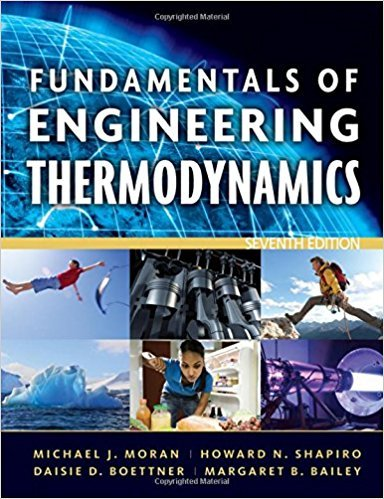×

# Using Entropy Data and ConceptsOne-tenth kmol of carbonISBN: 9780470495902 50

## Solution for problem 15P Chapter 6

Fundamentals of Engineering Thermodynamics | 7th Edition

• Textbook Solutions
• 2901 Step-by-step solutions solved by professors and subject experts
• Get 24/7 help from StudySoup virtual teaching assistantsFundamentals of Engineering Thermodynamics | 7th Edition

4 5 0 311 Reviews
31
5
Problem 15P

Using Entropy Data and Concepts

One-tenth kmol of carbon monoxide (CO) in a piston-cylinder assembly undergoes a process from p1 = 150 kPa, T1 = 300 K to p2 = 500 kPa, T2 = 370 K. For the process, W = −300 kJ. Employing the ideal gas model, determine

(a) the heat transfer, in kJ.

(b) the change in entropy, in kJ/K.

Show the process on a sketch of the T-s diagram.

Step-by-Step Solution:

Step 1 of 4</p>

In this problem, we have to find the heat transfer and the change in entropy and also sketch the  process on T-s diagram. The given data areStep 2 of 4</p>

The carbon monoxide modeled as an ideal gas where kinetic and potential energy effects can be ignored.

A) The energy balancewhereandFrom the table A-23 we getHence the heat transfer.

Step 3 of 4

Step 4 of 4

##### ISBN: 9780470495902

The answer to “Using Entropy Data and ConceptsOne-tenth kmol of carbon monoxide (CO) in a piston-cylinder assembly undergoes a process from p1 = 150 kPa, T1 = 300 K to p2 = 500 kPa, T2 = 370 K. For the process, W = ?300 kJ. Employing the ideal gas model, determine(a) the heat transfer, in kJ.________________(b) the change in entropy, in kJ/K.Show the process on a sketch of the T-s diagram.” is broken down into a number of easy to follow steps, and 68 words. This full solution covers the following key subjects: process, entropy, kpa, ideal, cylinder. This expansive textbook survival guide covers 14 chapters, and 1501 solutions. Since the solution to 15P from 6 chapter was answered, more than 737 students have viewed the full step-by-step answer. This textbook survival guide was created for the textbook: Fundamentals of Engineering Thermodynamics, edition: 7. The full step-by-step solution to problem: 15P from chapter: 6 was answered by , our top Engineering and Tech solution expert on 07/20/17, 09:01AM. Fundamentals of Engineering Thermodynamics was written by and is associated to the ISBN: 9780470495902.

Unlock Textbook Solution

Using Entropy Data and ConceptsOne-tenth kmol of carbon

×